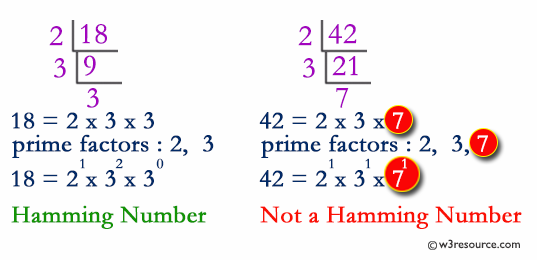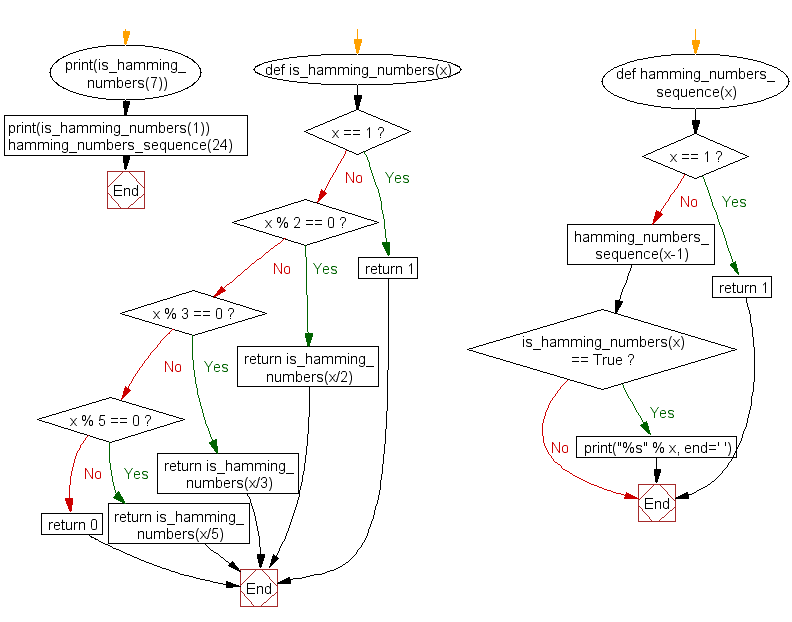﻿ Python: Get the Hamming numbers upto a given numbers also check whether a given number is a Hamming number - w3resource# Python Challenges: Get the Hamming numbers upto a given numbers also check whether a given number is a Hamming number

## Python Challenges - 1: Exercise-25 with Solution

Write a Python program to get the Hamming numbers upto a given numbers also check whether a given number is a Hamming number.

Hamming numbers are numbers of the form
H = 2i x 3j x 5k
Where i, j, k ≥ 0
The sequence of Hamming numbers 1, 2, 3, 4, 5, 6, 8, 9, 10, 12, 15, 16, 18, 20, 24, 25, 27. . . consists of all numbers of the form 2i.3j.5k where i, j and k are non-negative integers.

Explanation:Sample Solution:-

Python Code:

``````def is_hamming_numbers(x):
if x == 1:
return 1
if x % 2 == 0:
return is_hamming_numbers(x/2)
if x % 3 == 0:
return is_hamming_numbers(x/3)
if x % 5 == 0:
return is_hamming_numbers(x/5)
return 0

def hamming_numbers_sequence(x):
if x == 1:
return 1
hamming_numbers_sequence(x-1)
if is_hamming_numbers(x) == True:
print("%s" % x, end=' ')

print(is_hamming_numbers(7))
print(is_hamming_numbers(1))

hamming_numbers_sequence(24)
print()
```
```

Sample Output:

```0
1
2 3 4 5 6 8 9 10 12 15 16 18 20 24
```

Flowchart:## Visualize Python code execution:

The following tool visualize what the computer is doing step-by-step as it executes the said program:

Python Code Editor:

What is the difficulty level of this exercise?

Test your Python skills with w3resource's quiz

﻿

## Python: Tips of the Day

Getting the last element of a list:

some_list[-1] is the shortest and most Pythonic.

In fact, you can do much more with this syntax. The some_list[-n] syntax gets the nth-to-last element. So some_list[-1] gets the last element, some_list[-2] gets the second to last, etc, all the way down to some_list[-len(some_list)], which gives you the first element.

You can also set list elements in this way. For instance:

```>>> some_list = [1, 2, 3]
>>> some_list[-1] = 5 # Set the last element
>>> some_list[-2] = 3 # Set the second to last element
>>> some_list
[1, 3, 5]
```

Note that getting a list item by index will raise an IndexError if the expected item doesn't exist. This means that some_list[-1] will raise an exception if some_list is empty, because an empty list can't have a last element.

Ref: https://bit.ly/3d8TfFP